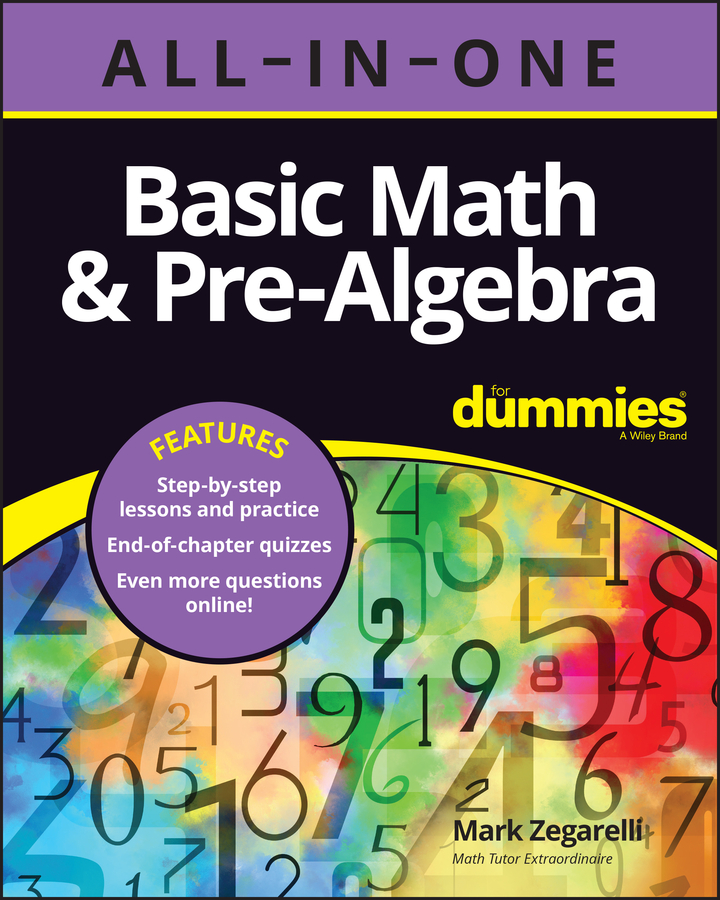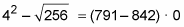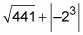##### Basic Math & Pre-Algebra All-in-One For Dummies (+ Chapter Quizzes Online)The ideas of equality, expressions, and evaluation are vital concepts when working with equations. An equation is a mathematical statement that tells you that two things have the same value — in other words, it’s a statement with an equal sign. The equation is one of the most important concepts in mathematics because it allows you to boil down a bunch of complicated information into a single number.

Mathematic equations come in lots of varieties, with the two most common being arithmetic and algebraic equations.

## Three properties of equality

The three properties of equality are called reflexivity, symmetry, and transitivity:

• Reflexivity says that everything is equal to itself. For example,

1 = 1

23 = 23

1,000,007 = 1,000,007

• Symmetry says that you can switch around the order in which things are equal. For example,

4 · 5 = 20, so 20 = 4 · 5

• Transitivity says that if something is equal to two other things, then those two other things are equal to each other. For example,

3 + 1 = 4 and 4 = 2 · 2, so 3 + 1 = 2 · 2

Because equality has all three of these properties, mathematicians call equality an equivalence relation.

Here are a few examples of simple arithmetic equations:

2 + 2 = 4

3 · 4 = 12

20 ÷ 2 = 10

And here are a few examples of more-complicated arithmetic equations:

1,000 – 1 – 1 – 1 = 997

(1 · 1) + (2 · 2) = 5## What is an expression?

An expression is any string of mathematical symbols that can be placed on one side of an equation. Here are a few examples of simple expressions:

2 + 2

–17 + (–1)

14 ÷ 7

And here are a few examples of more-complicated expressions:

(88 – 23) ÷ 13

100 + 2 – 3 · 17## How to evaluate expressions

At the root of the word evaluation is the word value. In other words, when you evaluate something, you find its value. Evaluating an expression is also referred to as simplifying, solving, or finding the value of an expression. The words may change, but the idea is the same — boiling a string of numbers and math symbols down to a single number.

When you evaluate an arithmetic expression, you simplify it to a single numerical value — that is, you find the number that it’s equal to. For example, evaluate the following arithmetic expression:

7 · 5

How? Simplify it to a single number:

35

## How do you connect equality, expressions, and evaluation?

The Three E’s — equality, expressions, and evaluation — are all connected. Evaluation allows you to take an expression containing more than one number and reduce it down to a single number. Then, you can make an equation, using an equal sign to connect the expression and the number. For example, here’s an expression containing four numbers:

1 + 2 + 3 + 4

When you evaluate it, you reduce it down to a single number:

10

And now, you can make an equation by connecting the expression and the number with an equal sign:

1 + 2 + 3 + 4 = 10